# Lower Triangular Matrix by Row-Major Mapping

## Lower Triangular Matrix by Row-Major Mapping in C and C++:

In this article, I am going to discuss Lower Triangular Matrix by Row-Major Mapping in C and C++ Language with Examples. Please read our previous article, where we discussed Diagonal Matrix in C and C++ Language with Examples.

##### Lower Triangular Matrix by Row-Major Mapping:

A lower triangular matrix is a square matrix in which the lower triangular part of a matrix is non-zero elements and the upper triangular part is all zeros and none of them is non-zero.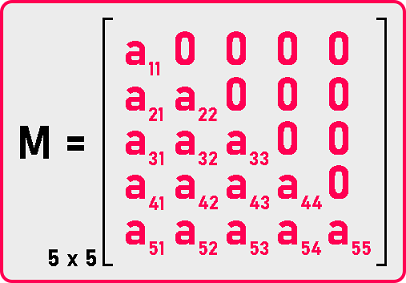The set of non-zero elements are forming a triangle. Now let us define this and see which elements are zero which are non-zero. Let us represent column numbers with ‘j’ and row numbers with ‘i’.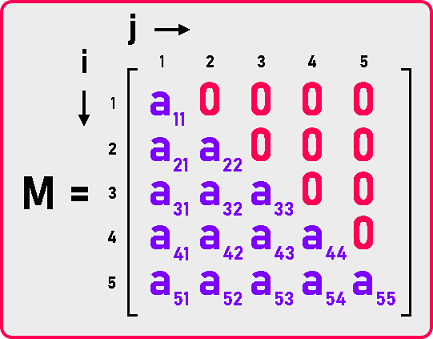If we check all the non-zero indices, the ‘j’ value is smaller or equal to the ‘i’ value. So, for non-zero elements ‘i’ value is always greater and for zero elements, the ‘i’ value is smaller. It means if the row number is less than the column number (i < j) then the element must be zero. We can define the lower triangular matrix as: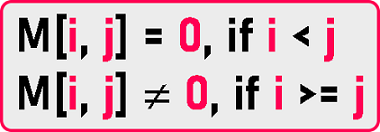Now let us observe in the matrix of 5×5, a total of 25 elements are there.

Then how many elements are non-zero?

1st row is having 1 non-zero element.

2nd row is having 2 non-zero elements.

5th row is having 5 non-zero elements.

For any n x n matrix, it will be: 1 + 2 + … + n. Means n (n + 1) / 2 elements are non-zero. In a matrix of n x n, total n2 elements will be there and n (n + 1) / 2 are non-zero elements. So, number of zero element is: Number of zero elements = n2 – n (n + 1) / 2 = n (n-1) / 2

The dimension of the matrix is 5 but the last number of zeros is 4. Therefore, total number of zeroes are n (n-1) / 2.

Now let us discuss representing this lower triangle of matrices in our program. So, when we want to use them in the program, we want to avoid storing zeros so that we can save memory space as well as we can save time in processing zero elements. Then for storing non-zero elements, how much space do we need?

= n (n + 1) / 2 = 5 (5 + 1) 2 = 15

That is a total of 15 spaces we need to store non-zero elements. Let us see how it can be represented. So, we will take an array of the size of 15 and we will learn how to store these elements.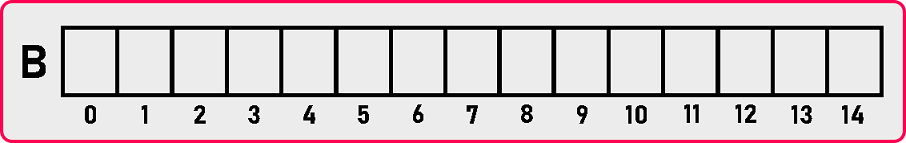Here we have created an array of size 15. Now we can store all the non-zero elements in this array.

Let’s know how to store these elements.

1st method is we can store them row by row.

2nd method is we can store them column by column also.

We follow the row by row method we can call it the row-major method.

##### Lower Triangular Matrix using Row Major Method:

Let’s fill the array row by row:

###### 1st row: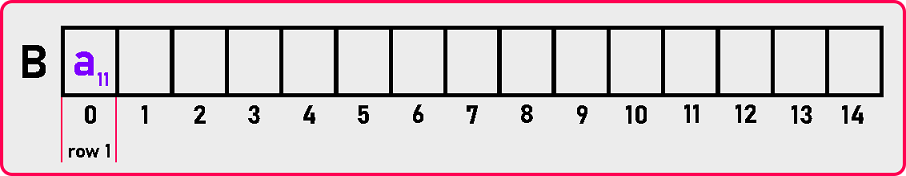###### 2nd row: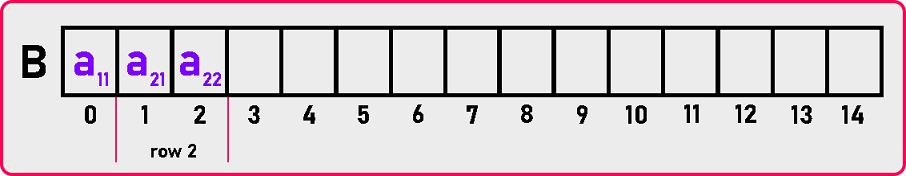###### 3rd row: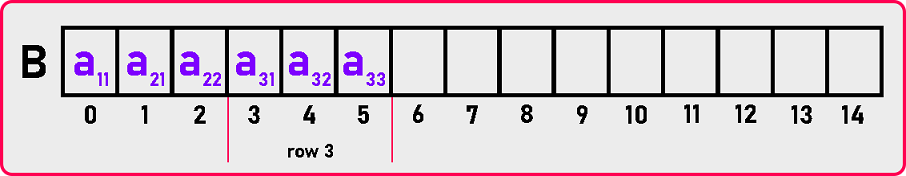###### 4th row: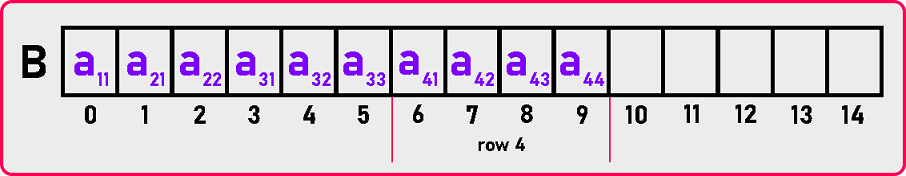###### 5th row: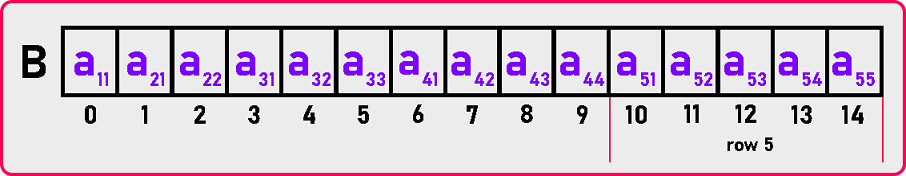Note: In the matrix, we have started indexing from 1 onwards but in C or C++ or any other programming language, indexing starts from 0 onwards. So, we have to take care of it.

Here we have simply stored the elements row by row but is there any formula for mapping the elements from lower triangular matrix to single dimension array. Let us come up with some formula so that we can access a specific element. Let us see how we can build up a formula.

Suppose we want to access an element that is a42 which is present in the 4th row. And a42 is present at the 7th index in the array. So, we have to move 7 indices to reach the a42 elements.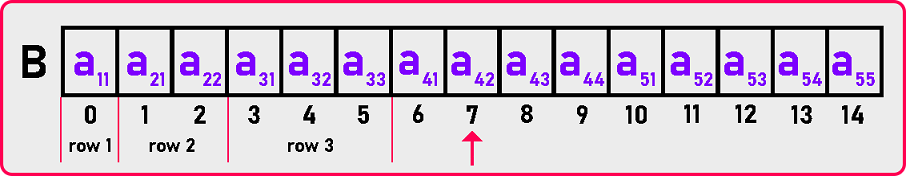To reach the given element, we have to skip 3 rows as you can see in the diagram. We can write the above thing as: Index (M ) = 1 + 2 + 3 +

The above equation is not complete. After skipping 3 rows, how many elements do we have to move so that we will point on the given element.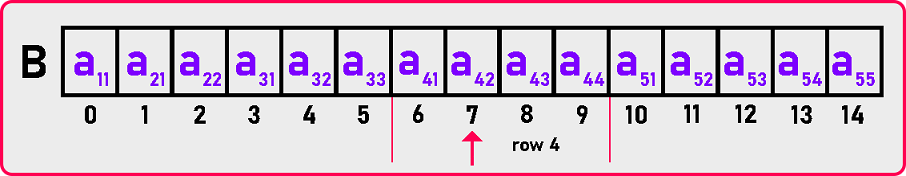As seen in the above diagram, we have to move 1 element that is a41.

Now complete the above equation as:

Index (M ) = 1 + 2 + 3 + 1

Index (M ) = 7

Now we got 7, which is the index of our given element. So yes, we got the index.

We did the sum of n natural numbers for skipping the rows. But where do we have to calculate? As seen in the above example, the value of row number or ‘i’ was 4 so we have calculated – 1+2+3. Means till 3 we have calculated the sum of n natural number. It means we have to calculate for i – 1 value (1 less than the value of i). Now the formula for the sum of n natural number in terms of i is:

= i (i – 1) / 2

After skipping the rows, we add the number of elements we have to skip to reach the given element. In our example, the value of ‘j’ was 2, but we add 1 element, which means we have to add j-1. So, the formula for any row number and column number is: Index (M [i][j]) = [i * (i – 1) / 2] + (j-1)

Now let see the code part:

##### Lower Triangular Matrix by Row-Major Mapping Code in C Language:
```#include <stdio.h>
#include <stdlib.h>

struct Matrix
{
int *B;
int n;
};

void Set (struct Matrix *m, int i, int j, int y)
{
if (i >= j)
m->B[i * (i - 1) / 2 + j - 1] = y;
}

int Get (struct Matrix m, int i, int j)
{
if (i >= j)
return m.B[i * (i - 1) / 2 + j - 1];
else
return 0;
}

void Display (struct Matrix m)
{
int i, j;
printf ("\nMatrix is: \n");
for (i = 1; i <= m.n; i++)
{
for (j = 1; j <= m.n; j++)
{
if (i >= j)
printf ("%d ", m.B[i * (i - 1) / 2 + j - 1]);
else
printf ("0 ");
}
printf ("\n");
}
}

int main ()
{
struct Matrix M;
int i, j, y;

printf ("Enter Dimension of Matrix: ");
scanf ("%d", &M.n);
M.B = (int *) malloc (M.n * (M.n + 1) / 2 * sizeof (int));

printf ("Enter all the elements of the matrix:\n");
for (i = 1; i <= M.n; i++)
{
for (j = 1; j <= M.n; j++)
{
scanf ("%d", &y);
Set (&M, i, j, y);
}
}

Display (M);
}
```
###### Output: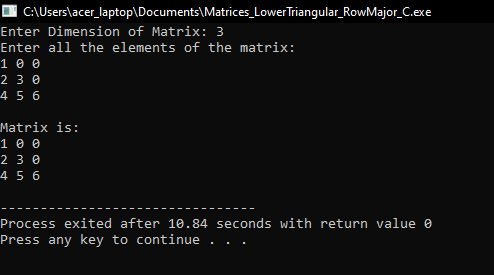##### Lower Triangular Matrix by Row-Major Mapping Code in C++ Language:
```#include <iostream>
using namespace std;

class LowerTriangularMatrix
{
private:
int *B;
int n;

public:
LowerTriangularMatrix ()
{
n = 3;
B = new int[3 * (3 + 1) / 2];
}

LowerTriangularMatrix (int n)
{
this->n = n;
B = new int[n * (n + 1) / 2];
}

~LowerTriangularMatrix ()
{
delete[]B;
}

int GetDimension ()
{
return n;
}

void Set (int i, int j, int y);
int Get (int i, int j);
void Display ();
};

void LowerTriangularMatrix::Set (int i, int j, int y)
{
if (i >= j)
B[i * (i - 1) / 2 + j - 1] = y;
}

int LowerTriangularMatrix::Get (int i, int j)
{
if (i >= j)
return B[i * (i - 1) / 2 + j - 1];
return 0;
}

void LowerTriangularMatrix::Display ()
{
cout << "\nMatrix is: " << endl;
for (int i = 1; i <= n; i++)
{
for (int j = 1; j <= n; j++)
{
if (i >= j)
cout << B[i * (i - 1) / 2 + j - 1] << " ";
else
cout << "0 ";
}
cout << endl;
}
}

int main ()
{
int d;
cout << "Enter Dimensions: ";
cin >> d;

LowerTriangularMatrix lm (d);

int x;
cout << "Enter All Elements: " << endl;
for (int i = 1; i <= d; i++)
{
for (int j = 1; j <= d; j++)
{
cin >> x;
lm.Set (i, j, x);
}
}

lm.Display ();
return 0;
}
```
###### Output: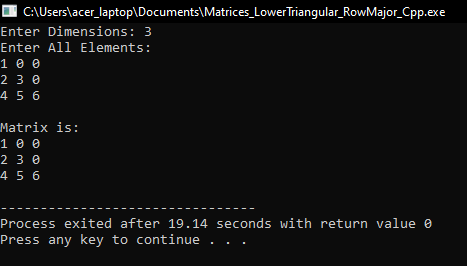In the next article, I am going to discuss Lower Triangular Matrix Column-Major Mapping in C and C++ Language with Examples. Here, in this article, I try to explain Lower Triangular Matrix Row-Major Mapping in C and C++ Language with Examples and I hope you enjoy this Lower Triangular Matrix Row-Major Mapping in C and C++ Language with Examples article.